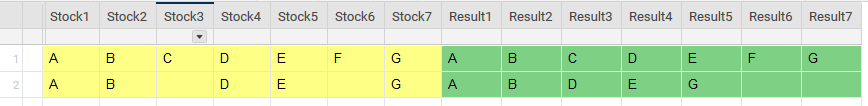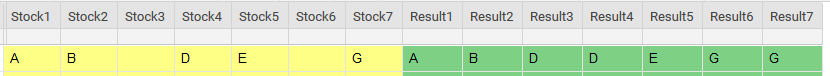# Genius Level IF (?) Formula

Does such a formula exist to acheive the below?

If I edit the Stock columns in Row 1, I want the Result columns to appear as Row 2 (i.e. data shifted to the left eliminating blank cells).

I'm nearly there with this formula, but it's not quite correct.

=IF([Stock1]1 <> "", [Stock1]1, IF([Stock2]1 <> "", [Stock2]1, IF([Stock3]1 <> "", [Stock3]1, IF([Stock4]1 <> "", [Stock4]1, IF([Stock5]1 <> "", [Stock5]1, IF([Stock6]1 <> "", [Stock6]1, IF([Stock7]1 <> "", [Stock7]1)))))))